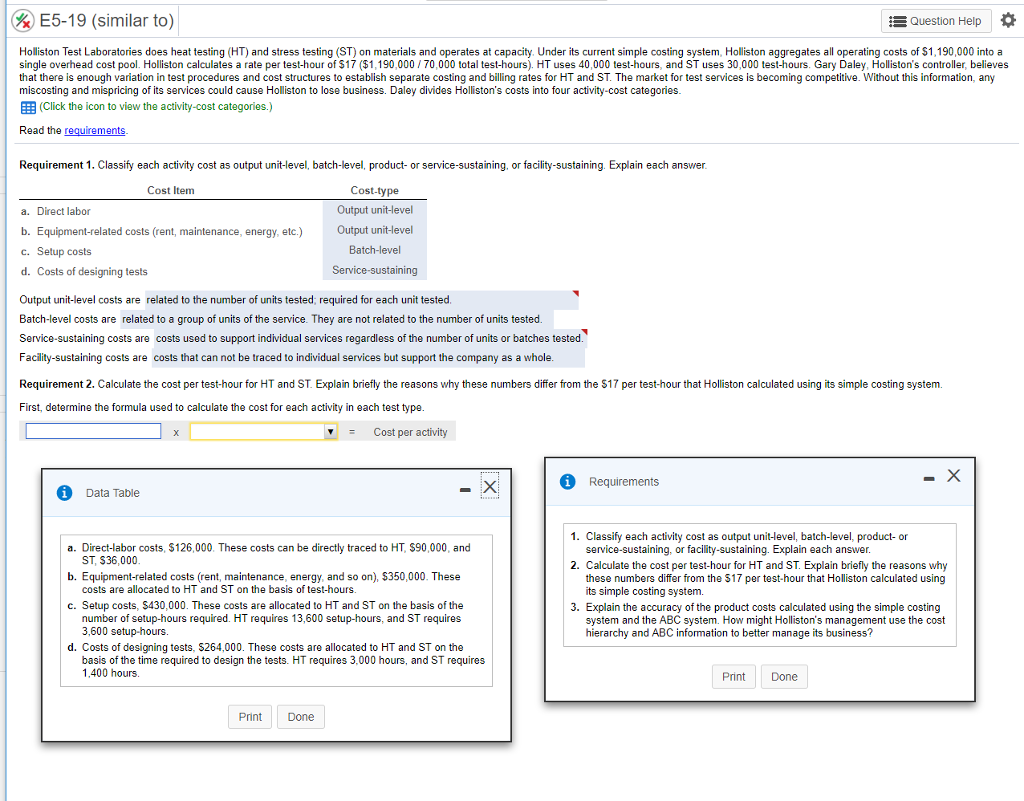# Question & Answer: & E5-19 (similar to) Question Help Holliston Test Laboratories does heat testing (HT) and stress testing (ST) on materials and operates at capacit…..& E5-19 (similar to) Question Help Holliston Test Laboratories does heat testing (HT) and stress testing (ST) on materials and operates at capacity. Under its current simple costing system, Holliston aggregates all operating costs of \$1,190,000 into a single overhead cost pool. Holliston calculates a rate per test-hour of \$17 (\$1,190,000/70,000 total test-hours). HT uses 40,000 test-hours, and ST uses 30,000 test-hours. Gary Daley, Holliston’s controller, believes that there is enough variation in test procedures and cost structures to establish separate costing and billing rates for HT and ST. The market for test services is becoming competitive. Without this information, any miscosting and mispricing of its services could cause Holliston to lose business. Daley divides Holliston’s costs into four activity-cost categories (Click the icon to view the activity-cost categories.) Read the requirements Requirement 1. Classify each activity cost as output unit-level, batch-level, product- or service-sustaining, or facility-sustaining. Explain each answer. Cost Item Cost-type Output unit-level Output unit-level Batch-level Service-sustaining a. Direct labor b. Equipment-related costs (rent, maintenance, energy, etc.) c. Setup costs d. Costs of designing tests Output unit-level costs are related to the number of units tested; required for each unit tested Batch-level costs are related to a group of units of the service. They are not related to the number of units tested. Service-sustaining costs are costs used to support individual services regardless of the number of units or batches tested. Facility-sustaining costs are costs that can not be traced to individual services but support the company as a whole Requirement 2. Calculate the cost per test-hour for HT and ST. Explain briefly the reasons why these numbers differ from the \$17 per test-hour that Holliston calculated using its simple costing system. First, determine the formula used to calculate the cost for each activity in each test type. Cost per activity Requirements Data Table 1. Classify each activity cost as output unit-level, batch-level, product- or a. Direct-labor costs, \$126,000. These costs can be directly traced to HT, \$90,000, and b. Equipment-related costs (rent, maintenance, energy, and so on), \$350,000. These c. Setup costs, \$430,000. These costs are allocated to HT and ST on the basis of the service-sustaining, or facility-sustaining. Explain each answer. ST, S36,000 2. Calculate the cost per test-hour for HT and ST. Explain briefly the reasons why these numbers differ from the S17 per test-hour that Holliston calculated using its simple costing system. costs are allocated to HT and ST on the basis of test-hours. 3. Explain the accuracy of the product costs calculated using the simple costing number of setup-hours required. HT requires 13,600 setup-hours, and ST requires 3,600 setup-hours. system and the ABC system. How might Holliston’s management use the cost hierarchy and ABC information to better manage its business? d. Costs of designing tests, \$264,000. These costs are allocated to HT and ST on the basis of the time required to design the tests. HT requires 3,000 hours, and ST requires 1400 hours PrintDone Print Done

Don't use plagiarized sources. Get Your Custom Essay on
Question & Answer: & E5-19 (similar to) Question Help Holliston Test Laboratories does heat testing (HT) and stress testing (ST) on materials and operates at capacit…..
GET AN ESSAY WRITTEN FOR YOU FROM AS LOW AS \$13/PAGE

Solution

Requirement 1 is given, which is correct.

Requirement 2.

Formula:

activity cost/assigned activity base=cost per activity

Cost allocation:

 HT total cost(1) HT per hour cost(2)=(1)/40000 ST total cost(3) ST per hour (4)=(3)/30000 Direct labour costs(given) \$90,000 \$2.25 (90000/40000) \$36,000 \$1.20 (36000/30000) Equipment related cost (350,000/(40000+30000)=\$5 200,000 (40000×\$5) \$5 \$150,000 (30000×\$5) \$5 Set up costs (430,000/(13600+3600)=\$25 \$340,000 (13600×\$25) \$8.50 \$90,000 (3600×\$25) \$3.00 Cost of designing tests (264000/(3000+1400)=\$60 \$180,000 (3000×\$60) \$4.59 \$84,000 (1400×\$60) \$2.80 Total costs \$820,000 \$20.50 \$370,000 \$12.33

Simple costing(\$17) undercosts HT(\$20.50) and over costs ST(\$12.33). Reason for this :

HT uses direct labour, setup and designing resources per hour more than ST. In simple costing it is assumed that testing cost per hour remain same for both the tests.

3.

ABC system better calculated the product costs because it identifies all the activities undertaken while performing the tests and recognize the level of the cost hierarchy at which costs vary.

Management can use the information to make better pricing and product mix decision. For example : decision to increase the price of HT and decrease the price of ST test.